# Fall 2021 Final Exam

Instructor(s): Janine Tiefenbruck, Suraj Rampure

This exam was administered remotely via Gradescope. The exam was open-internet, and students were able to use Jupyter Notebooks. They had 3 hours to work on it.

Welcome to the Final Exam! In honor of the (at the time) brand-new Comic-Con Museum that just opened at Balboa Park here in San Diego, this exam will contain questions about various museums and zoos around the world.

In this question, we’ll work with the DataFrame art_museums, which contains the name, city, number of visitors in 2019, and rank (based on number of visitors) for the 100 most visited art museums in 2019. The first few rows of art_museums are shown below.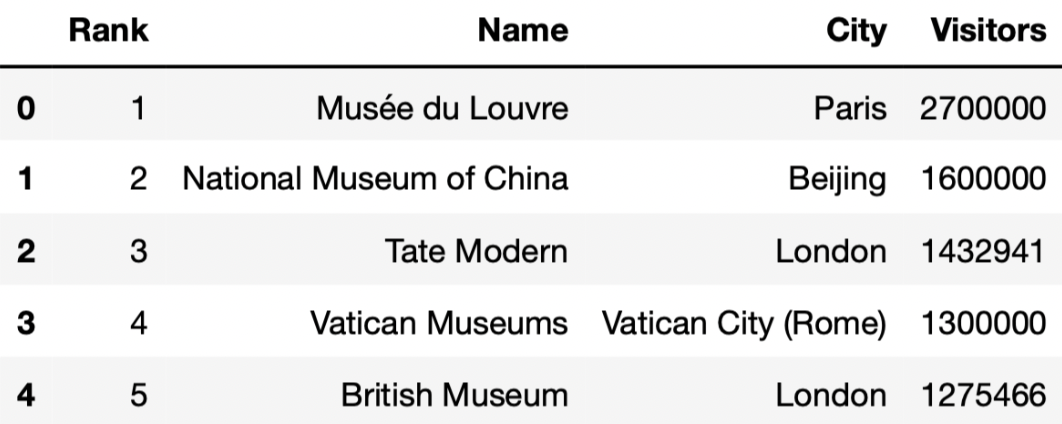Tip: Open this page in another tab, so that it is easy to refer to this data description as you work through the exam.

## Problem 1

### Problem 1.1

Which of the following blocks of code correctly assigns random_art_museums to an array of the names of 10 art museums, randomly selected without replacement from those in art_museums? Select all that apply.

Option 1:

def get_10(df):
return np.array(df.sample(10).get('Name'))

random_art_museums = get_10(art_museums)

Option 2:

def get_10(art_museums):
return np.array(art_museums.sample(10).get('Name'))

random_art_museums = get_10(art_museums)

Option 3:

def get_10(art_museums):
random_art_museums = np.array(art_museums.sample(10).get('Name'))

random_art_museums = get_10(art_museums)

Option 4:

def get_10():
return np.array(art_museums.sample(10).get('Name'))

random_art_museums = get_10()

Option 5:

random_art_museums = np.array([])

def get_10():
random_art_museums = np.array(art_museums.sample(10).get('Name'))
return random_art_museums

get_10()
• Option 1

• Option 2

• Option 3

• Option 4

• Option 5

• None of the above

Answers: Option 1, Option 2, and Option 4

Note that if df is a DataFrame, then df.sample(10) is a DataFrame containing 10 randomly selected rows in df. With that in mind, let’s look at all of our options.

• Option 1: This implementation of get_10 takes in a DataFrame df and returns an array containing 10 randomly selected values in df’s 'Name' column. After defining get_10, we assign random_art_museums to the result of calling get_10(art_museums). This assigns random_art_museums as intended, so Option 1 is correct.
• Option 2: This option is functionally the same as Option 1, so it is also correct. The only difference between Option 2 and Option 1 is that Option 2 uses the parameter name art_museums and Option 1 uses the parameter name df (both in the def line and in the function body); this does not change the behavior of get_10 or the lines afterward.
• Option 3: get_10 here does not return anything! So, get_10(art_museums) evaluates to None (which means “nothing” in Python), and random_art_museums is also None, meaning Option 3 is incorrect.
• Option 4: At first, it may appear that this option is wrong, as get_10 does not take in any inputs. However, the body of get_10 contains a reference to the DataFrame art_museums, which is ultimately where we want to sample from. As a result, get_10 does indeed return an array containing 10 randomly selected museum names, and random_art_museums = get_10() correctly assigns random_art_museums to this array, so Option 4 is correct.
• Option 5: Here, get_10 returns the correct array. However, outside of the function, random_art_museums is never assigned to the output of get_10. (The variable name random_art_museums inside the function has nothing to do with the array defined before and outside the function.) As a result, after running the line get_10() at the bottom of the code block, random_art_museums is still an empty array, and as such, Option 5 is incorrect.

##### Difficulty: ⭐️⭐️

The average score on this problem was 85%.

### Problem 1.2

London has the most art museums in the top 100 of any city in the world. The most visited art museum in London is 'Tate Modern'.

Which of the following blocks of code correctly assigns best_in_london to 'Tate Modern'? Select all that apply.

Option 1:

def most_common(df, col):
return df.groupby(col).count().sort_values(by='Rank', ascending=False).index

def most_visited(df, col, value):
return df[df.get(col)==value].sort_values(by='Visitors', ascending=False).get('Name').iloc

best_in_london = most_visited(art_museums, 'City', most_common(art_museums, 'City'))

Option 2:

def most_common(df, col):
print(df.groupby(col).count().sort_values(by='Rank', ascending=False).index)

def most_visited(df, col, value):
print(df[df.get(col)==value].sort_values(by='Visitors', ascending=False).get('Name').iloc)

best_in_london = most_visited(art_museums, 'City', most_common(art_museums, 'City'))

Option 3:

def most_common(df, col):
return df.groupby(col).count().sort_values(by='Rank', ascending=False).index

def most_visited(df, col, value):
print(df[df.get(col)==value].sort_values(by='Visitors', ascending=False).get('Name').iloc)

best_in_london = most_visited(art_museums, 'City', most_common(art_museums, 'City'))
• Option 1

• Option 2

• Option 3

• None of the above

At a glance, it may seem like there’s a lot of reading to do to answer the question. However, it turns out that all 3 options follow similar logic; the difference is in their use of print and return statements. Whenever we want to “save” the output of a function to a variable name or use it in another function, we need to return somewhere within our function. Only Option 1 contains a return statement in both most_common and most_visited, so it is the only correct option.

Let’s walk through the logic of Option 1 (which we don’t necessarily need to do to answer the problem, but we should in order to enhance our understanding):

• First, we use most_common to find the city with the most art museums. most_common does this by grouping the input DataFrame df (art_museums, in this case) by 'City' and using the .count() method to find the number of rows per 'City'. Note that when using .count(), all columns in the aggregated DataFrame will contain the same information, so it doesn’t matter which column you use to extract the counts per group. After sorting by one of these columns ('Rank', in this case) in decreasing order, most_common takes the first value in the index, which will be the name of the 'City' with the most art museums. This is London, i.e. most_common(art_museums, 'City') evaluates to 'London' in Option 1 (in Option 2, it evaluates to None, since most_common there doesn’t return anything).
• Then, we use most_visited to find the museum with the most visitors in the city with the most museums. This is achieved by keeping only the rows of the input DataFrame df (again, art_museums in this case) where the value in the col ('City') column is value (most_common(art_museums, 'City'), or 'London'). Now that we only have information for museums in London, we can sort by 'Visitors' to find the most visited such museum, and take the first value from the resulting 'Name' column. While all 3 options follow this logic, only Option 1 returns the desired value, and so only Option 1 assigns best_in_london correctly. (Even if Option 2’s most_visited used return instead of print, it still wouldn’t work, since Option 2’s most_common also uses print instead of return).

##### Difficulty: ⭐️⭐️

The average score on this problem was 86%.

## Problem 2

In this question, we’ll keep working with the art_museums DataFrame.

### Problem 2.1

(Remember to keep the data description from the top of the exam open in another tab!)

'Tate Modern' is the most popular art museum in London. But what’s the most popular art museum in each city?

It turns out that there’s no way to answer this easily using the tools that you know about so far. To help, we’ve created a new Series method, .last(). If s is a Series, s.last() returns the last element of s (i.e. the element at the very end of s). .last() works with .groupby, too (just like .mean() and .count()).

Fill in the blanks so that the code below correctly assigns best_per_city to a DataFrame with one row per city, that describes the name, number of visitors, and rank of the most visited art museum in each city. best_per_city should be sorted in decreasing order of number of visitors. The first few rows of best_per_city are shown below.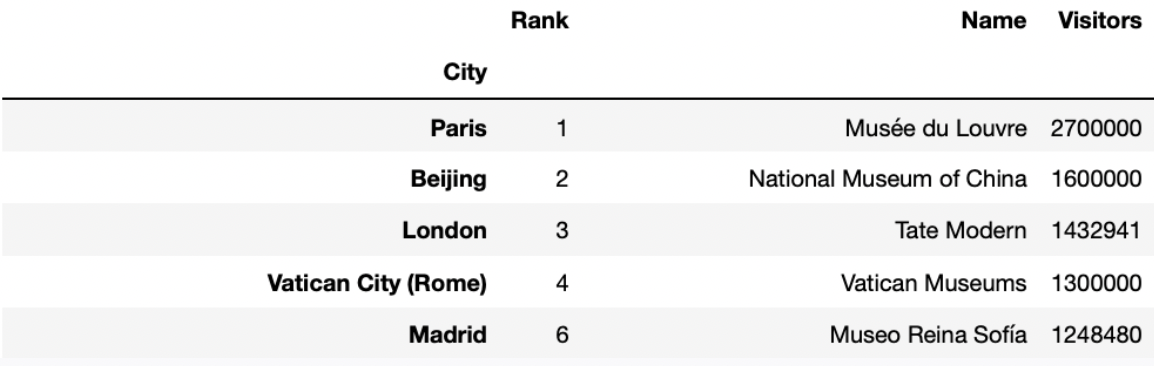best_per_city = __(a)__.groupby(__(b)__).last().__(c)__
1. What goes in blank (a)?
2. What goes in blank (b)?
3. What goes in blank (c)?

1. art_museums.sort_values('Visitors', ascending=True)
2. 'City'
3. sort_values('Visitors', ascending=False)

Let’s take a look at the completed implementation.

best_per_city = art_museums.sort_values('Visitors', ascending=True).groupby('City').last().sort_values('Visitors', ascending=False)

We first sort the row in art_museums by the number of 'Visitors' in ascending order. Then goupby('City'), so that we have one row per city. Recall, we need an aggregation method after using groupby(). In this question, we use last() to only keep the one last row for each city. Since in blank (a) we have sorted the rows by 'Visitors', so last() keeps the row that contains the name of the most visited museum in that city. At last we sort this new DataFrame by 'Visitors' in descending order to fulfill the question’s requirement.

##### Difficulty: ⭐️⭐️⭐️

The average score on this problem was 65%.

### Problem 2.2

Assume you’ve defined best_per_city correctly.

Which of the following options evaluates to the number of visitors to the most visited art museum in Amsterdam? Select all that apply.

• best_per_city.get('Visitors').loc['Amsterdam']

• best_per_city[best_per_city.index == 'Amsterdam'].get('Visitors').iloc

• best_per_city[best_per_city.index == 'Amsterdam'].get('Visitors').iloc[-1]

• best_per_city[best_per_city.index == 'Amsterdam'].get('Visitors').loc['Amsterdam']

• None of the above

Answer: best_per_city.get('Visitors').loc['Amsterdam'], best_per_city[best_per_city.index == 'Amsterdam'].get('Visitors').iloc, best_per_city[best_per_city.index == 'Amsterdam'].get('Visitors').iloc[-1], best_per_city[best_per_city.index == 'Amsterdam'].get('Visitors').loc['Amsterdam'] (Select all except “None of the above”)

best_per_city.get('Visitors').loc['Amsterdam'] We first use .get(column_name) to get a series with number of visitors to the most visited art museum, and then locate the number of visitors to the most visited art museum in Amsterdam using .loc[index] since we have "City" as index.

best_per_city[best_per_city.index == 'Amsterdam'].get('Visitors').iloc We first query the best_per_city to only include the DataFrame with one row with index 'Amsterdam'. Then, we get the 'Visitors' column of this DataFrame. Finally, we use iloc to access the first and the only value in this column.

best_per_city[best_per_city.index == 'Amsterdam'].get('Visitors').iloc[-1] We first query the best_per_city to only include the DataFrame with one row with index 'Amsterdam'. Then, we get the 'Visitors' column of this DataFrame. Finally, we use iloc[-1] to access the last and the only value in this column.

best_per_city[best_per_city.index == 'Amsterdam'].get('Visitors').loc['Amsterdam'] We first query the best_per_city to only include the DataFrame with one row with index 'Amsterdam'. Then, we get the 'Visitors' column of this DataFrame. Finally, we use loc['Amsterdam'] to access the value in this column with index 'Amsterdam'.

##### Difficulty: ⭐️⭐️

The average score on this problem was 84%.

## Problem 3

The table below shows the average amount of revenue from different sources for art museums in 2003 and 2013.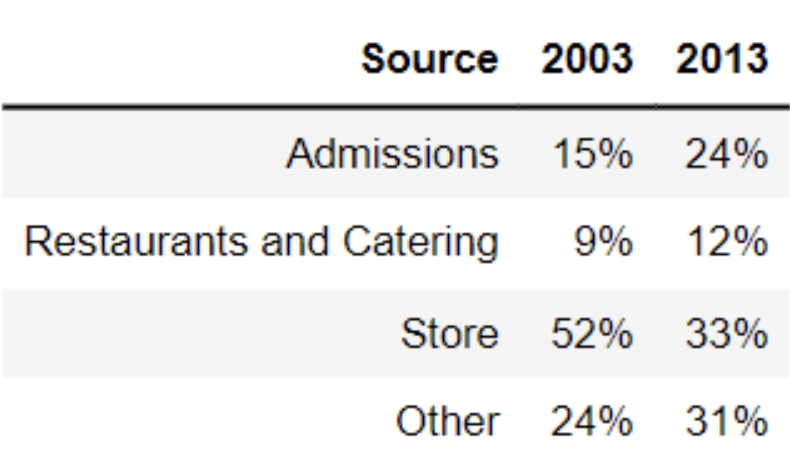### Problem 3.1

What is the total variation distance between the distribution of revenue sources in 2003 and the distribution of revenue sources in 2013? Give your answer as a proportion (i.e. a decimal between 0 and 1), not a percentage. Round your answer to three decimal places.

Recall, the total variation distance (TVD) is the sum of the absolute differences in proportions, divided by 2. The absolute differences in proportions for each source are as follows:

• Admissions: |0.15 - 0.24| = 0.09
• Restaurants and Catering: |0.09 - 0.12| = 0.03
• Store: |0.52 - 0.33| = 0.19
• Other: |0.24 - 0.31| = 0.07

Then, we have

\text{TVD} = \frac{1}{2} (0.09 + 0.03 + 0.19 + 0.07) = 0.19

##### Difficulty: ⭐️

The average score on this problem was 95%.

### Problem 3.2

Which type of visualization would be best suited for comparing the two distributions in the table?

• Scatter plot

• Line plot

• Overlaid histogram

• Overlaid bar chart

A scatter plot visualizes the relationship between two numerical variables. In this problem, we only have to visualize the distribution of a categorical variable.

A line plot shows trends in numerical variables over time. In this problem, we only have categorical variables. Moreover, when it says over time, it is suitable for plotting change in multiple years (e.g. 2001, 2002, 2003, … , 2013), or even with data of days. In this question, we only want to compare the distribution of 2003 and 2013, this makes the line plot not useful. In addition, if you try to draw a line plot for this question, you will find the line plot fails to visualize distribution (e.g. the idea of 15%, 9%, 52%, and 24% add up to 100%).

An overlaid graph is useful in this question since this visualizes comparison between the two distributions.

However, an overlaid histogram is not useful in this problem. The key reason is the differences between a histogram and a bar chart.

Bar Chart: Space between the bars; 1 categorical axis, 1 numerical axis; order does not matter
Histogram: No space between the bars; intervals on axis; 2 numerical axes; order matters

In the question, we are plotting 2003 and 2013 distributions of four categories (Admissions, Restaurants and Catering, Store, and Other). Thus, an overlaid bar chart is more appropriate.

##### Difficulty: ⭐️⭐️⭐️

The average score on this problem was 74%.

### Problem 3.3

Notably, there was an economic recession in 2008-2009. Which of the following can we conclude was an effect of the recession?

• The increase in revenue from admissions, as more people were visiting museums.

• The decline in revenue from museum stores, as people had less money to spend.

• The decline in total revenue, as fewer people were visiting museums.

• None of the above

Since we are only given the distribution of the revenue, and have no information about the amount of revenue in 2003 and 2013, we cannot conclude how the revenue has changed from 2003 to 2013 after the recession.

For instance, if the total revenue in 2003 was 100 billion USD and the total revenue in 2013 was 50 billion USD, revenue from admissions in 2003 was 100 * 15% = 15 billion USD, and revenue from admissions in 2003 was 50 * 24% = 12 billion USD. In this case, we will have 15 > 12, the revenue from admissions has declined rather than increased (As stated by ‘The increase in revenue from admissions, as more people were visiting museums.’). Similarly, since we don’t know the total revenue in 2003 and 2013, we cannot conclude ‘The decline in revenue from museum stores, as people had less money to spend.’ or ‘The decline in total revenue, as fewer people were visiting museums.’

##### Difficulty: ⭐️⭐️⭐️

The average score on this problem was 72%.

## Problem 4

### Problem 4.1

The Museum of Natural History has a large collection of dinosaur bones, and they know the approximate year each bone is from. They want to use this sample of dinosaur bones to estimate the total number of years that dinosaurs lived on Earth. We’ll make the assumption that the sample is a uniform random sample from the population of all dinosaur bones. Which statistic below will give the best estimate of the population parameter?

• sample sum

• sample max - sample min

• 2 \cdot (sample mean - sample min)

• 2 \cdot (sample max - sample mean)

• 2 \cdot sample mean

• 2 \cdot sample median

Answer: sample max - sample min

Our goal is to estimate the total number of years that dinosaurs lived on Earth. In other words, we want to know the range of time that dinosaurs lived on Earth, and by definition range = biggest value - smallest value. By using “sample max - sample min”, we calculate the difference between the earliest and the latest dinosaur bones in this uniform random sample, which helps us to estimate the population range.

##### Difficulty: ⭐️⭐️⭐️

The average score on this problem was 52%.

### Problem 4.2

The curator at the Museum of Natural History, who happens to have taken a data science course in college, points out that the estimate of the parameter obtained from this sample could certainly have come out differently, if the museum had started with a different sample of bones. The curator suggests trying to understand the distribution of the sample statistic. Which of the following would be an appropriate way to create that distribution?

• bootstrapping the original sample

• using the Centrual Limit Theorem

• both bootstrapping and the Central Limit Theorem

• neither bootstrapping nor the Central Limit Theorem

Answer: neither bootstrapping nor the Central Limit Theorem

Recall, the Central Limit Theorem (CLT) says that the probability distribution of the sum or average of a large random sample drawn with replacement will be roughly normal, regardless of the distribution of the population from which the sample is drawn. Thus, the theorem only applies when our sample statistics is sum or average, while in this question, our statistics is range, so CLT does not apply.

Bootstrapping is a valid technique for estimating measures of central tendency, e.g. the population mean, median, standard deviation, etc. It doesn’t work well in estimating extreme or sensitive values, like the population maximum or minimum. Since the statistic we’re trying to estimate is the difference between the population maximum and population minimum, bootstrapping is not appropriate.

##### Difficulty: ⭐️⭐️⭐️⭐️⭐️

The average score on this problem was 20%.

## Problem 5

### Problem 5.1

Now, the Museum of Natural History wants to know how many visitors they have in a year. However, their computer systems are rather archaic and so they aren’t able to keep track of the number of tickets sold for an entire year. Instead, they randomly select five days in the year, and keep track of the number of visitors on those days. Let’s call these numbers v_1, v_2, v_3, v_4, and v_5.

Which of the following is the best estimate the number of visitors for the entire year?

• v_1 + v_2 + v_3 + v_4 + v_5

• \frac{5}{365}\cdot(v_1 + v_2 + v_3 + v_4 + v_5)

• \frac{365}{5}\cdot(v_1 + v_2 + v_3 + v_4 + v_5)

• 365\cdot v_3

Answer: \frac{365}{5}\cdot(v_1 + v_2 + v_3 + v_4 + v_5)

Our sample is the number of visitors on the five days, and our population is the number of visitors in all 365 days.

First, we calculate the sample mean, the average number of visitors in the 5 days, which is m = \frac{1}{5}\cdot(v_1 + v_2 + v_3 + v_4 + v_5). We use this statistic to estimate the population mean, the average number of visitors in this year.

Then, we use the estimated population mean to calculate the estimated pupulation sum, so we multiply the number of days in a year (365) with the estimated population mean. We get 365 m = \frac{365}{5}\cdot(v_1 + v_2 + v_3 + v_4 + v_5)

##### Difficulty: ⭐️

The average score on this problem was 92%.

### Problem 5.2

Now we’re interested in predicting the admission cost of a museum based on its number of visitors. Suppose:

• admission cost and number of visitors are linearly associated with a correlation coefficient of 0.25,

• the number of visitors at the Museum of Natural History is six standard deviations below average,

• the average cost of museum admission is 15 dollars, and

• the standard deviation of admission cost is 3 dollars.

What would the regression line predict for the admission cost (in dollars) at the Museum of Natural History? Give your answer as a number without any units, rounded to three decimal places.

Recall, we can make predictions in standard units with the following formula

\text{predicted}\ y_{su} = r \cdot x_{su}

We’re given that the correlation coefficient, r, between visitors and admission cost is 0.25. Here, we’re using the number of visitors (x) to predict admission cost (y). Given that the number of visitors at the Museum of Natural History is 6 standard deviations below average,

\text{predicted}\ y_{su} = r \cdot x_{su} = 0.25 \cdot -6 = -1.5

We then compute y, which is the admission cost (in dollars) at the Museum of Natural History.

\begin{align*} y_{su} = \frac{y- \text{Mean of } y}{\text{SD of } y}\\ -1.5 &= \frac{y-15}{3} \\ -1.5 \cdot 3 &= y-15\\ -4.5 + 15 &= y\\ y &= \boxed{10.5} \end{align*}

So, the regression line predicts that the admission cost at the Museum of Natural History is \$10.50.

##### Difficulty: ⭐️⭐️⭐️

The average score on this problem was 62%.

## Problem 6

Researchers from the San Diego Zoo, located within Balboa Park, collected physical measurements of several species of penguins in a region of Antarctica.

One piece of information they tracked for each of 330 penguins was its mass in grams. The average penguin mass is 4200 grams, and the standard deviation is 840 grams.

### Problem 6.1

Consider the histogram of mass below.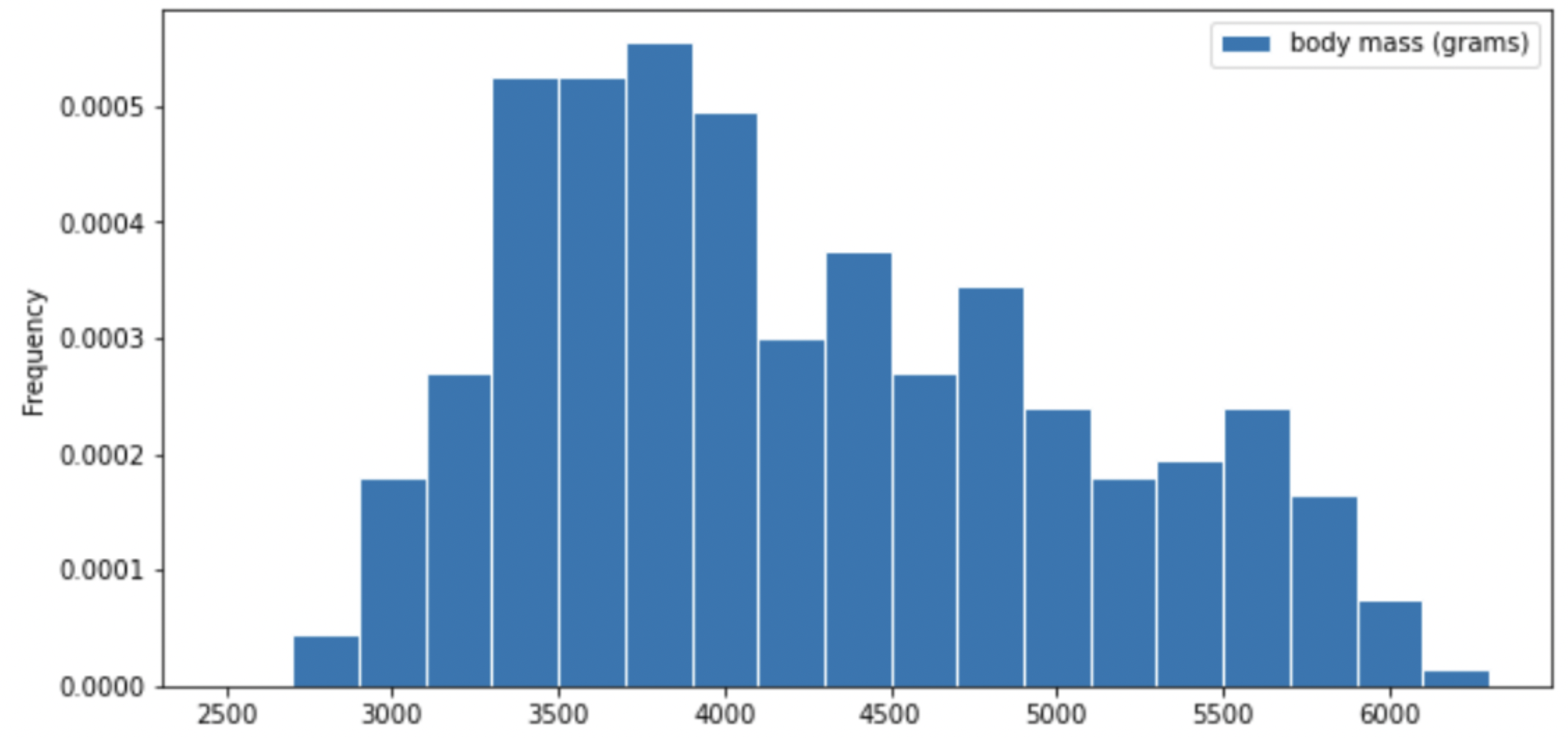Select the true statement below.

• The median mass of penguins is larger than the average mass of penguins

• The median mass of penguins is roughly equal to the average mass of penguins (within 50 grams)

• The median mass of penguins is less than the average mass of penguins

• It is impossible to determine the relationship between the median and average mass of penguins just by looking at the above histogram

Answer: The median mass of penguins is less than the average mass of penguins

This is a distribution that is skewed to the right, so mean is greater than median.

##### Difficulty: ⭐️⭐️

The average score on this problem was 87%.

### Problem 6.2

Which of the following is a valid conclusion that we can draw solely from the histogram above?

• The number of penguins with a mass of exactly 3500 grams is greater than the number of penguins with a mass of exactly 5500 grams.

• The number of penguins with a mass of at most 3500 grams is greater than the number of penguins with a mass of at least 5500 grams.

• There is an odd number of penguins in the dataset.

• The number penguins with a mass of exactly 4000 grams is greater than zero.

• None of the above.

Answer: The number of penguins with a mass of at most 3500 grams is greater than the number of penguins with a mass of at least 5500 grams.

Recall, a histogram has intervals on the axis, so we cannot know the frequency of an exact value. Thus, we cannot conclude statements 1, 3, 4. Since the frequency of an exact value is unknown, for statement 3, it is possible that all numbers we have in this distribution are even. Although in the graph, we are only given frequency rather than number, we can justify statement 2 by comparing the area in the left side of 3500, and the area in the right side of 5500. You can either estimate by visually comparing the areas of both parts or compute the area sum of both sides by estimating the bars’ height and windth.

##### Difficulty: ⭐️⭐️

The average score on this problem was 89%.

### Problem 6.3

For your convenience, we show the histogram of mass again below.Recall, there are 330 penguins in our dataset. Their average mass is 4200 grams, and the standard deviation of mass is 840 grams.

Per Chebyshev’s inequality, at least what percentage of penguins have a mass between 3276 grams and 5124 grams? Input your answer as a percentage between 0 and 100, without the % symbol. Round to three decimal places.

Recall, Chebyshev’s inequality states that No matter what the shape of the distribution is, the proportion of values in the range “average ± z SDs” is at least 1 - \frac{1}{z^2}.

To approach the problem, we’ll start by converting 3276 grams and 5124 grams to standard units. Doing so yields \frac{3276 - 4200}{840} = -1.1, similarly, \frac{5124 - 4200}{840} = 1.1. This means that 3276 is 1.1 standard deviations below the mean, and 5124 is 1.1 standard deviations above the mean. Thus, we are calculating the proportion of values in the range “average ± 1.1 SDs”.

When z = 1.1, we have 1 - \frac{1}{z^2} = 1 - \frac{1}{1.1^2} \approx 0.173553719, which as a percentage rounded to three decimal places is 17.355\%.

##### Difficulty: ⭐️⭐️

The average score on this problem was 76%.

### Problem 6.4

Per Chebyshev’s inequality, at least what percentage of penguins have a mass between 1680 grams and 5880 grams?

• 50%

• 55.5%

• 65.25%

• 68%

• 75%

• 88.8%

• 95%

Recall: proportion with z SDs of the mean

Percent in Range All Distributions (via Chebyshev’s Inequality) Normal Distributions
\text{average} \pm 1 \ \text{SD} \geq 0\% \approx 68\%
\text{average} \pm 2\text{SDs} \geq 75\% \approx 95\%
\text{average} \pm 3\text{SDs} \geq 88\% \approx 99.73\%

To approach the problem, we’ll start by converting 3276 grams and 5124 grams to standard units. Doing so yields \frac{1680 - 4200}{840} = -3, similarly, \frac{5880 - 4200}{840} = 2. This means that 1680 is 3 standard deviations below the mean, and 5880 is 2 standard deviations above the mean.

Proportion of values in [-3 SUs, 2 SUs] >= Proportion of values in [-2 SUs, 2 SUs] >= 75% (Since we cannot assume that the distribution is normal, we look at the All Distributions (via Chebyshev’s Inequality) column for proportion).

Thus, at least 75% of the penguins have a mass between 1680 grams and 5880 grams.

##### Difficulty: ⭐️⭐️⭐️

The average score on this problem was 72%.

### Problem 6.5

The distribution of mass in grams is not roughly normal. Is the distribution of mass in standard units roughly normal?

• Yes

• No

• Impossible to tell

The shape of the distribution does not change since we are scaling the x values for all data.

##### Difficulty: ⭐️⭐️⭐️

The average score on this problem was 60%.

### Problem 6.6

Suppose all 330 penguin body masses (in grams) that the researchers collected are stored in an array called masses. We’d like to estimate the probability that two different randomly selected penguins from our dataset have body masses within 50 grams of one another (including a difference of exactly 50 grams). Fill in the missing pieces of the simulation below so that the function estimate_prob_within_50g returns an estimate for this probability.

def estimate_prob_within_50g():
num_reps = 10000
within_50g_count = 0
for i in np.arange(num_reps):
two_penguins = np.random.choice(__(a)__)
if __(b)__:
within_50g_count = within_50g_count + 1
return within_50g_count / num_reps

What goes in blank (a)? What goes in blank (b)?

Answer: (a) masses, 2, replace=False (b) abs(two_penguins - two_penguins)<=50

1. Recall, np.random.choice( ) can have three parameters array, n, replace=False, and returns n elements from the array at random, without replacement. We are randomly choosing 2 different penguins from the masses array, so we are using np.random.choice( ) without replacement.
2. We want to count the number of pairs of penguins that have body masses difference within 50 grams, so we are using the index to access the two penguins generated from two_penguins and calculating their absolute difference with abs(). And in this if condition, we only want to have penguins with absolute difference less than or equal to 50, so we write a <= condition to justify whether the generated pairs of penguins fulfill this requirement.

##### Difficulty: ⭐️⭐️

The average score on this problem was 84%.

### Problem 6.7

Recall, there are 330 penguins in our dataset. Their average mass is 4200 grams, and the standard deviation of mass is 840 grams. Assume that the 330 penguins in our dataset are a random sample from the population of all penguins in Antarctica. Our sample gives us one estimate of the population mean.

To better estimate the population mean, we bootstrapped our sample and plotted a histogram of the resample means, then took the middle 68 percent of those values to get a confidence interval. Which option below shows the histogram of the resample means and the confidence interval we found?

Option 1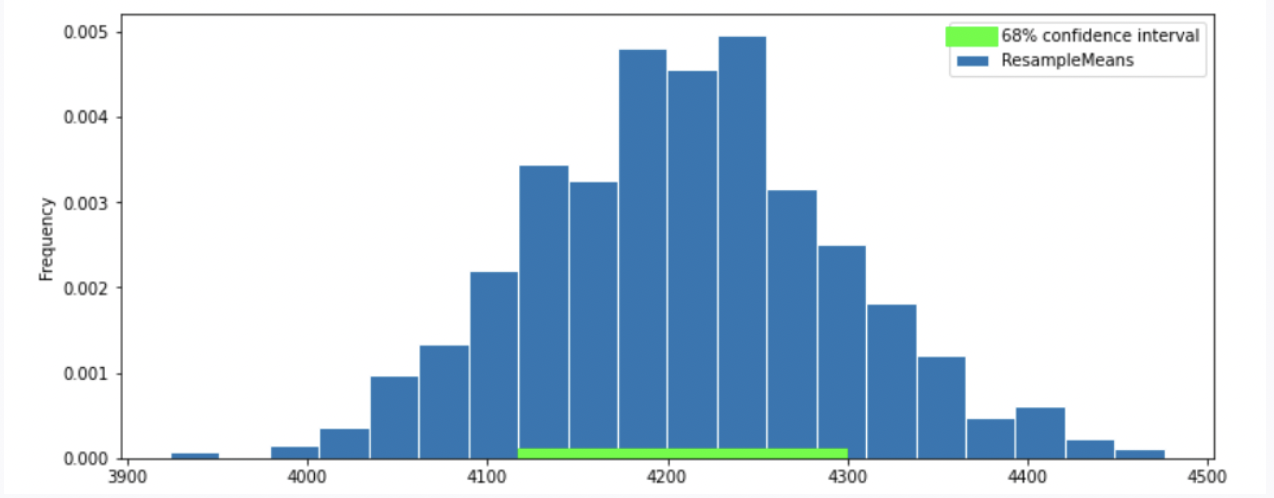Option 2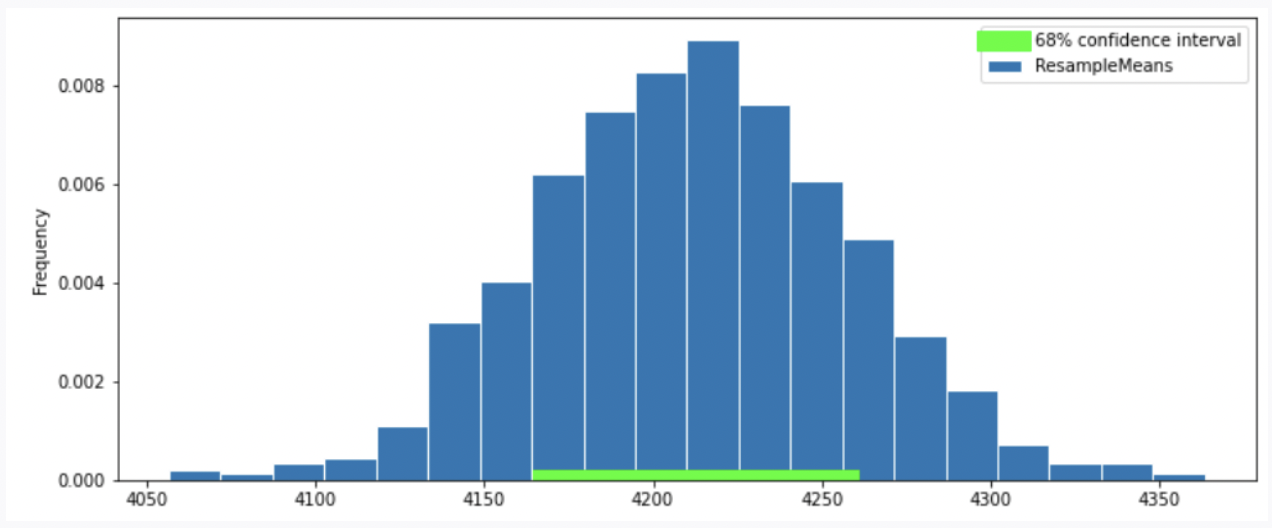Option 3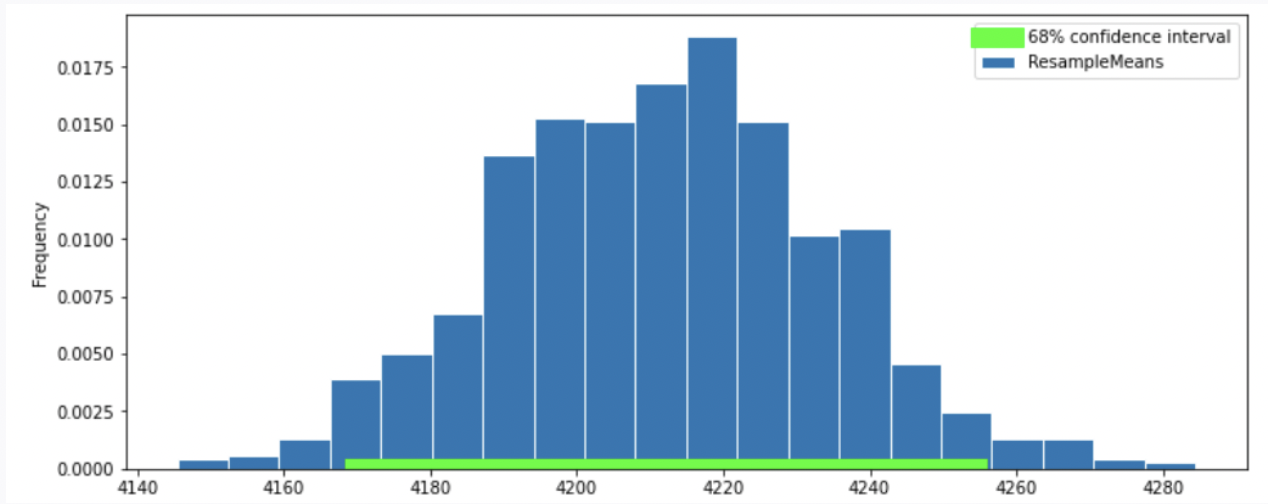Option 4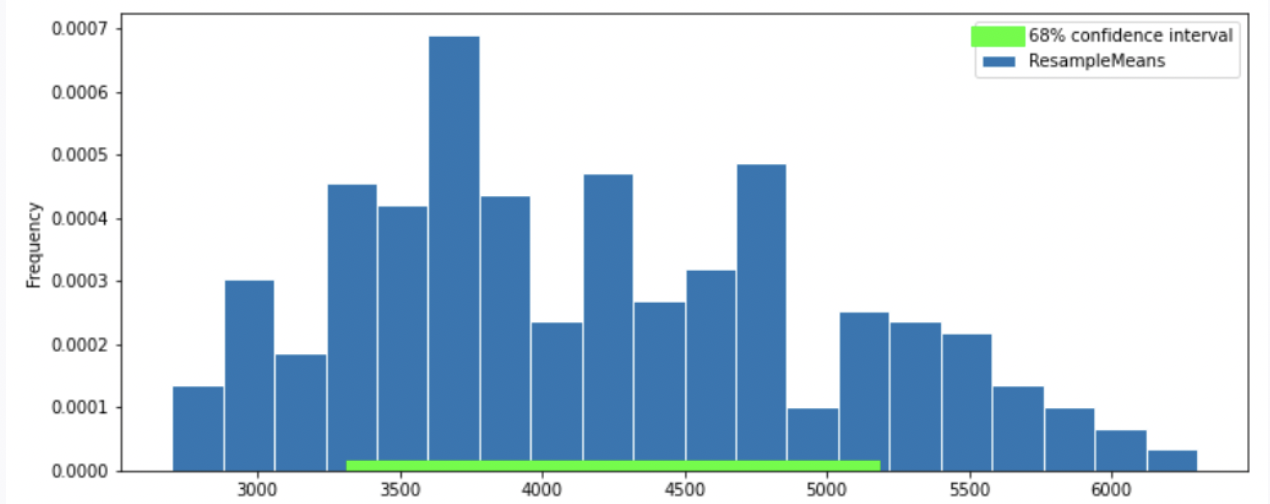Recall, according to the Central Limit Theorem (CLT), the probability distribution of the sum or mean of a large random sample drawn with replacement will be roughly normal, regardless of the distribution of the population from which the sample is drawn.

Thus, our graph should have a normal distribution. We eliminate Option 4.

Recall that the standard normal curve has inflection points at z = +-1, which is 68% proportion of a normal distribution.(inflection point is where a curve goes from “opening down” to “opening up”) Since we have a confidence intervel of 68% in this question, by looking at the inflection point, we can eliminate Option 3

To compute the SD of the sample mean’s distribution, when we don’t know the population’s SD, we can use the sample’s SD (840): \text{SD of Distribution of Possible Sample Means} \approx \frac{\text{Sample SD}}{\sqrt{\text{sample size}}} = \frac{840}{\sqrt{330}} \approx 46.24

Recall: proportion with z SDs of the mean

Percent in Range All Distributions (via Chebyshev’s Inequality) Normal Distributions
\text{average} \pm 1 \ \text{SD} \geq 0\% \approx 68\%
\text{average} \pm 2\text{SDs} \geq 75\% \approx 95\%
\text{average} \pm 3\text{SDs} \geq 88\% \approx 99.73\%

In this question, we want 68% confidence interval, given that the distribution of sample mean is roughly normal, our CI should have range \text{sample mean} \pm 1 \ \text{SD}. Thus, the interval is approximately [4200-46.24 = 4153.76, 4200+46.24=4246.24]. We compare the 68% CI in Option 1, 2 and we choose Option 2 since it has a 68% CI with approximately the same interval.

##### Difficulty: ⭐️⭐️⭐️

The average score on this problem was 66%.

### Problem 6.8

Suppose boot_means is an array of the resampled means. Fill in the blanks below so that [left, right] is a 68% confidence interval for the true mean mass of penguins.

left = np.percentile(boot_means, __(a)__)
right = np.percentile(boot_means, __(b)__)
[left, right]

What goes in blank (a)? What goes in blank (b)?

Recall, np.percentile(array, p) computes the pth percentile of the numbers in array. To compute the 68% CI, we need to know the percentile of left tail and right tail.

left percentile = (1-0.68)/2 = (0.32)/2 = 0.16 so we have 16th percentile

right percentile = 1-((1-0.68)/2) = 1-((0.32)/2) = 1-0.16 = 0.84 so we have 84th percentile

##### Difficulty: ⭐️

The average score on this problem was 94%.

### Problem 6.9

Which of the following is a correct interpretation of this confidence interval? Select all that apply.

• There is an approximately 68% chance that mean weight of all penguins in Antarctica falls within the bounds of this confidence interval.

• Approximately 68% of penguin weights in our sample fall within the bounds of this confidence interval.

• Approximately 68% of penguin weights in the population fall within the bounds of this interval.

• If we created many confidence intervals using the same method, approximately 68% of them would contain the mean weight of all penguins in Antarctica.

• None of the above

Answer: Option 4 (If we created many confidence intervals using the same method, approximately 68% of them would contain the mean weight of all penguins in Antarctica.)

Recall, what a k% confidence level states is that approximately k% of the time, the intervals you create through this process will contain the true population parameter.

In this question, our population parameter is the mean weight of all penguins in Antarctica. So 86% of the time, the intervals you create through this process will contain the mean weight of all penguins in Antarctica. This is the same as Option 4. However, it will be false if we state it in the reverse order (Option 1) since our population parameter is already fixed.

##### Difficulty: ⭐️⭐️

The average score on this problem was 81%.

## Problem 7

Now let’s study the relationship between a penguin’s bill length (in millimeters) and mass (in grams). Suppose we’re given that

• bill length and body mass have a correlation coefficient of 0.55
• the average bill length is 44 mm and the standard deviation of bill lengths is 6 mm
• as before, the average body mass is 4200 grams and the standard deviation of body mass is 840 grams

### Problem 7.1

Which of the four scatter plots below describe the relationship between bill length and body mass, based on the information provided in the question?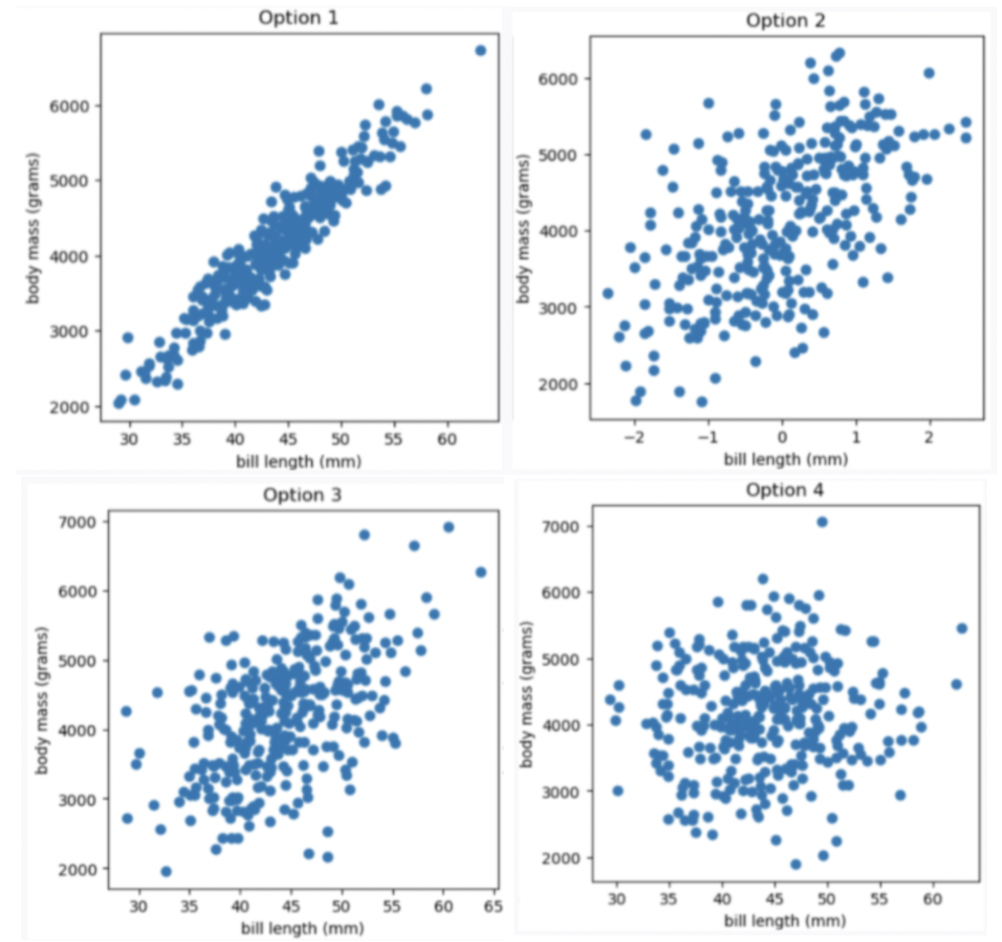• Option 1

• Option 2

• Option 3

• Option 4

Given the correlation coefficient is 0.55, bill length and body mass has a moderate positive correlation. We eliminate Option 1 (strong correlation) and Option 4 (weak correlation).

Given the average bill length is 44 mm, we expect our x-axis to have 44 at the middle, so we eliminate Option 2

##### Difficulty: ⭐️

The average score on this problem was 91%.

### Problem 7.2

Suppose we want to find the regression line that uses bill length, x, to predict body mass, y. The line is of the form y = mx +\ b. What are m and b?

What is m? Give your answer as a number without any units, rounded to three decimal places.

What is b? Give your answer as a number without units, rounded to three decimal places.

Answer: m = 77, b = 812

m = r \cdot \frac{\text{SD of }y }{\text{SD of }x} = 0.55 \cdot \frac{840}{6} = 77 b = \text{mean of }y - m \cdot \text{mean of }x = 4200-77 \cdot 44 = 812

##### Difficulty: ⭐️

The average score on this problem was 92%.

### Problem 7.3

What is the predicted body mass (in grams) of a penguin whose bill length is 44 mm? Give your answer as a number without any units, rounded to three decimal places.

y = mx\ +\ b = 77 \cdot 44 + 812 = 3388 +812 = 4200

##### Difficulty: ⭐️

The average score on this problem was 95%.

### Problem 7.4

A particular penguin had a predicted body mass of 6800 grams. What is that penguin’s bill length (in mm)? Give your answer as a number without any units, rounded to three decimal places.

In this question, we want to compute x value given y value y = mx\ +\ b y - b = mx \frac{y - b}{m} = x\ \ \text{(m is nonzero)} x = \frac{y - b}{m} = \frac{6800 - 812}{77} = \frac{5988}{77} \approx 77.766

##### Difficulty: ⭐️⭐️

The average score on this problem was 88%.

### Problem 7.5

Below is the residual plot for our regression line.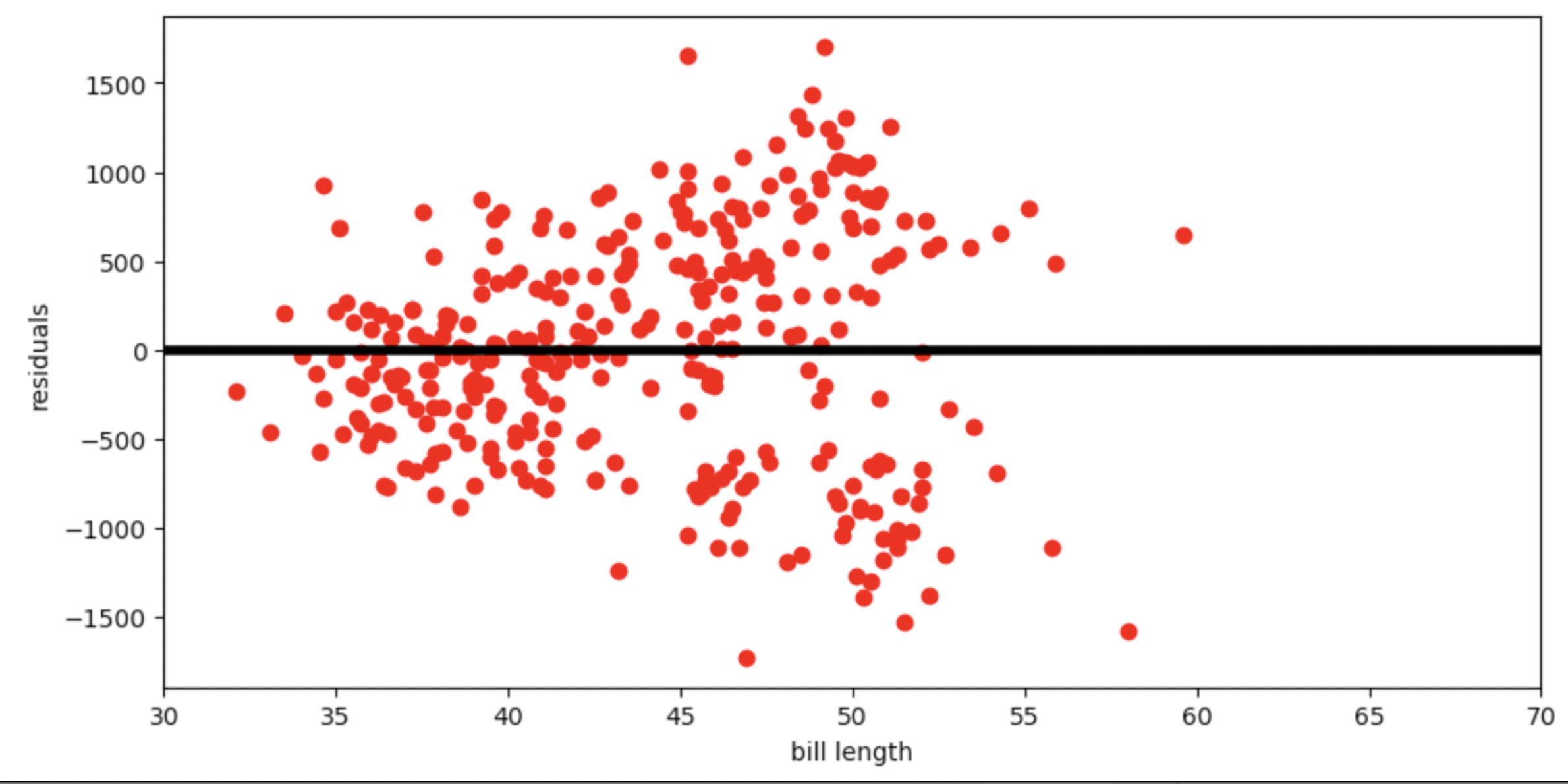Which of the following is a valid conclusion that we can draw solely from the residual plot above?

• For this dataset, there is another line with a lower root mean squared error

• The root mean squared error of the regression line is 0

• The accuracy of the regression line’s predictions depends on bill length

• The relationship between bill length and body mass is likely non-linear

• None of the above

Answer: The accuracy of the regression line’s predictions depends on bill length

The vertical spread in this residual plot is uneven, which implies that the regression line’s predictions aren’t equally accurate for all inputs. This doesn’t necessarily mean that fitting a nonlinear curve would be better. It just impacts how we interpret the regression line’s predictions.

##### Difficulty: ⭐️⭐️⭐️⭐️

The average score on this problem was 40%.

## Problem 8

Each individual penguin in our dataset is of a certain species (Adelie, Chinstrap, or Gentoo) and comes from a particular island in Antarctica (Biscoe, Dream, or Torgerson). There are 330 penguins in our dataset, grouped by species and island as shown below.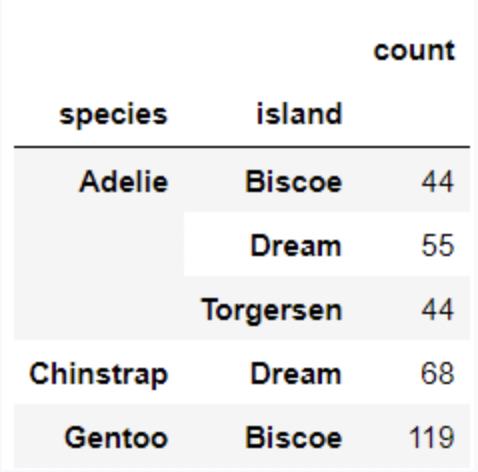Suppose we pick one of these 330 penguins, uniformly at random, and name it Chester.

### Problem 8.1

What is the probability that Chester comes from Dream island? Give your answer as a number between 0 and 1, rounded to three decimal places.

P(Chester comes from Dream island) = # of penguins in dream island / # of all penguins in the data = \frac{55+68}{330} \approx 0.373

##### Difficulty: ⭐️

The average score on this problem was 94%.

### Problem 8.2

If we know that Chester comes from Dream island, what is the probability that Chester is an Adelie penguin? Give your answer as a number between 0 and 1, rounded to three decimal places.

P(Chester is an Adelie penguin given that Chester comes from Dream island) = # of Adelie penguins from Dream island / # of penguins from Dream island = \frac{55}{55+68} \approx 0.447

##### Difficulty: ⭐️

The average score on this problem was 91%.

### Problem 8.3

If we know that Chester is not from Dream island, what is the probability that Chester is not an Adelie penguin? Give your answer as a number between 0 and 1, rounded to three decimal places.

Method 1

P(Chester is not an Adelie penguin given that Chester is not from Dream island) = # of penguins that are not Adelie penguins from islands other than Dream island / # of penguins in island other than Dream island = \frac{119\ \text{(eliminate all penguins that are Adelie or from Dream island, only Gentoo penguins from Biscoe are left)}}{44+44+119} \approx 0.575

Method 2

P(Chester is not an Adelie penguin given that Chester is not from Dream island) = 1- (# of penguins that are Adelie penguins from islands other than Dream island / # of penguins in island other than Dream island) = 1-\frac{44+44}{44+44+119} \approx 0.575

##### Difficulty: ⭐️⭐️

The average score on this problem was 85%.

## Problem 9

We’re now interested in investigating the differences between the masses of Adelie penguins and Chinstrap penguins. Specifically, our null hypothesis is that their masses are drawn from the same population distribution, and any observed differences are due to chance only.

Below, we have a snippet of working code for this hypothesis test, for a specific test statistic. Assume that adelie_chinstrap is a DataFrame of only Adelie and Chinstrap penguins, with just two columns – 'species' and 'mass'.

stats = np.array([])
num_reps = 500
for i in np.arange(num_reps):
# --- line (a) starts ---
# --- line (a) ends ---

# --- line (b) starts ---
# --- line (b) ends ---

grouped = with_shuffled.groupby('species').mean()

# --- line (c) starts ---
stat = grouped.get('mass').iloc - grouped.get('mass').iloc
# --- line (c) ends ---

stats = np.append(stats, stat)

### Problem 9.1

Which of the following statements best describe the procedure above?

• This is a standard hypothesis test, and our test statistic is the total variation distance between the distribution of Adelie masses and Chinstrap masses

• This is a standard hypothesis test, and our test statistic is the difference between the expected proportion of Adelie penguins and the proportion of Adelie penguins in our resample

• This is a permutation test, and our test statistic is the total variation distance between the distribution of Adelie masses and Chinstrap masses

• This is a permutation test, and our test statistic is the difference in the mean Adelie mass and mean Chinstrap mass

Answer: This is a permutation test, and our test statistic is the difference in the mean Adelie mass and mean Chinstrap mass (Option 4)

Recall, a permutation test helps us decide whether two random samples come from the same distribution. This test matches our goal of testing whether the masses of Adelie penguins and Chinstrap penguins are drawn from the same population distribution. The code above are also doing steps of a permutation test. In part (a), it shuffles 'species' and stores the shuffled series to shuffled. In part (b), it assign the shuffled series of values to 'species' column. Then, it uses grouped = with_shuffled.groupby('species').mean() to calculate the mean of each species. In part (c), it computes the difference between mean mass of the two species by first getting the 'mass' column and then accessing mean mass of each group (Adelie and Chinstrap) with positional index 0 and 1.

##### Difficulty: ⭐️

The average score on this problem was 98%.

### Problem 9.2

Currently, line (c) (marked with a comment) uses .iloc. Which of the following options compute the exact same statistic as line (c) currently does?

Option 1:

stat = grouped.get('mass').loc['Adelie'] - grouped.get('mass').loc['Chinstrap']

Option 2:

stat = grouped.get('mass').loc['Chinstrap'] - grouped.get('mass').loc['Adelie']
• Option 1 only

• Option 2 only

• Both options

• Neither option

We use df.get(column_name).iloc[positional_index] to access the value in a column with positional_index. Similarly, we use df.get(column_name).loc[index] to access value in a column with its index. Remember grouped is a DataFrame that groupby('species'), so we have species name 'Adelie' and 'Chinstrap' as index for grouped.

Option 2 is incorrect since it does subtraction in the reverse order which results in a different stat compared to line(c). Its output will be -1 \cdot stat. Recall, in grouped = with_shuffled.groupby('species').mean(), we use groupby() and since 'species' is a column with string values, our index will be sorted in alphabetical order. So, .iloc is 'Adelie' and .iloc is 'Chinstrap'.

##### Difficulty: ⭐️⭐️

The average score on this problem was 81%.

### Problem 9.3

Is it possible to re-write line (c) in a way that uses .iloc twice, without any other uses of .loc or .iloc?

• Yes, it’s possible

• No, it’s not possible

There are multiple ways to achieve this. For instance stat = grouped.get('mass').iloc - grouped.sort_index(ascending = False).get('mass').iloc.

##### Difficulty: ⭐️⭐️⭐️

The average score on this problem was 64%.

### Problem 9.4

For your convenience, we copy the code for the hypothesis test below.

stats = np.array([])
num_reps = 500
for i in np.arange(num_reps):
# --- line (a) starts ---
# --- line (a) ends ---

# --- line (b) starts ---
# --- line (b) ends ---

grouped = with_shuffled.groupby('species').mean()

# --- line (c) starts ---
stat = grouped.get('mass').iloc - grouped.get('mass').iloc
# --- line (c) ends ---

stats = np.append(stats, stat)

What would happen if we removed line (a), and replaced line (b) with

with_shuffled = adelie_chinstrap.sample(adelie_chinstrap.shape, replace=False)

• This would still run a valid hypothesis test

• This would not run a valid hypothesis test, as all values in the stats array would be exactly the same

• This would not run a valid hypothesis test, even though there would be several different values in the stats array

• This would not run a valid hypothesis test, as it would incorporate information about Gentoo penguins

Answer: This would not run a valid hypothesis test, as all values in the stats array would be exactly the same (Option 2)

Recall, DataFrame.sample(n, replace = False) (or DataFrame.sample(n) since replace = False is by default) returns a DataFrame by randomly sampling n rows from the DataFrame, without replacement. Since our n is adelie_chinstrap.shape, and we are sampling without replacement, we will get the exactly same Dataframe (though the order of rows may be different but the stats array would be exactly the same).

##### Difficulty: ⭐️⭐️

The average score on this problem was 87%.

### Problem 9.5

For your convenience, we copy the code for the hypothesis test below.

stats = np.array([])
num_reps = 500
for i in np.arange(num_reps):
# --- line (a) starts ---
# --- line (a) ends ---

# --- line (b) starts ---
# --- line (b) ends ---

grouped = with_shuffled.groupby('species').mean()

# --- line (c) starts ---
stat = grouped.get('mass').iloc - grouped.get('mass').iloc
# --- line (c) ends ---

stats = np.append(stats, stat)

What would happen if we removed line (a), and replaced line (b) with

with_shuffled = adelie_chinstrap.sample(adelie_chinstrap.shape, replace=True)

• This would still run a valid hypothesis test

• This would not run a valid hypothesis test, as all values in the stats array would be exactly the same

• This would not run a valid hypothesis test, even though there would be several different values in the stats array

• This would not run a valid hypothesis test, as it would incorporate information about Gentoo penguins

Answer: This would not run a valid hypothesis test, even though there would be several different values in the stats array (Option 3)

Recall, DataFrame.sample(n, replace = True) returns a new DataFrame by randomly sampling n rows from the DataFrame, with replacement. Since we are sampling with replacement, we will have a DataFrame which produces a stats array with some different values. However, recall, the key idea behind a permutation test is to shuffle the group labels. So, the above code does not meet this key requirement since we only want to shuffle the "species" column without changing the size of the two species. However, the code may change the size of the two species.

##### Difficulty: ⭐️⭐️⭐️

The average score on this problem was 66%.

### Problem 9.6

For your convenience, we copy the code for the hypothesis test below.

stats = np.array([])
num_reps = 500
for i in np.arange(num_reps):
# --- line (a) starts ---
# --- line (a) ends ---

# --- line (b) starts ---
# --- line (b) ends ---

grouped = with_shuffled.groupby('species').mean()

# --- line (c) starts ---
stat = grouped.get('mass').iloc - grouped.get('mass').iloc
# --- line (c) ends ---

stats = np.append(stats, stat)

What would happen if we replaced line (a) with

with_shuffled = adelie_chinstrap.assign(
)

and replaced line (b) with

with_shuffled = with_shuffled.assign(
)

• This would still run a valid hypothesis test

• This would not run a valid hypothesis test, as all values in the stats array would be exactly the same

• This would not run a valid hypothesis test, even though there would be several different values in the stats array

• This would not run a valid hypothesis test, as it would incorporate information about Gentoo penguins

Answer: This would still run a valid hypothesis test (Option 1)

Our goal for the permutation test is to randomly assign birth weights to groups, without changing group sizes. The above code shuffles 'species' and 'mass' columns and assigns them back to the DataFrame. This fulfills our goal.

##### Difficulty: ⭐️⭐️

The average score on this problem was 81%.

### Problem 9.7

Suppose we run the code for the hypothesis test and see the following empirical distribution for the test statistic. In red is the observed statistic.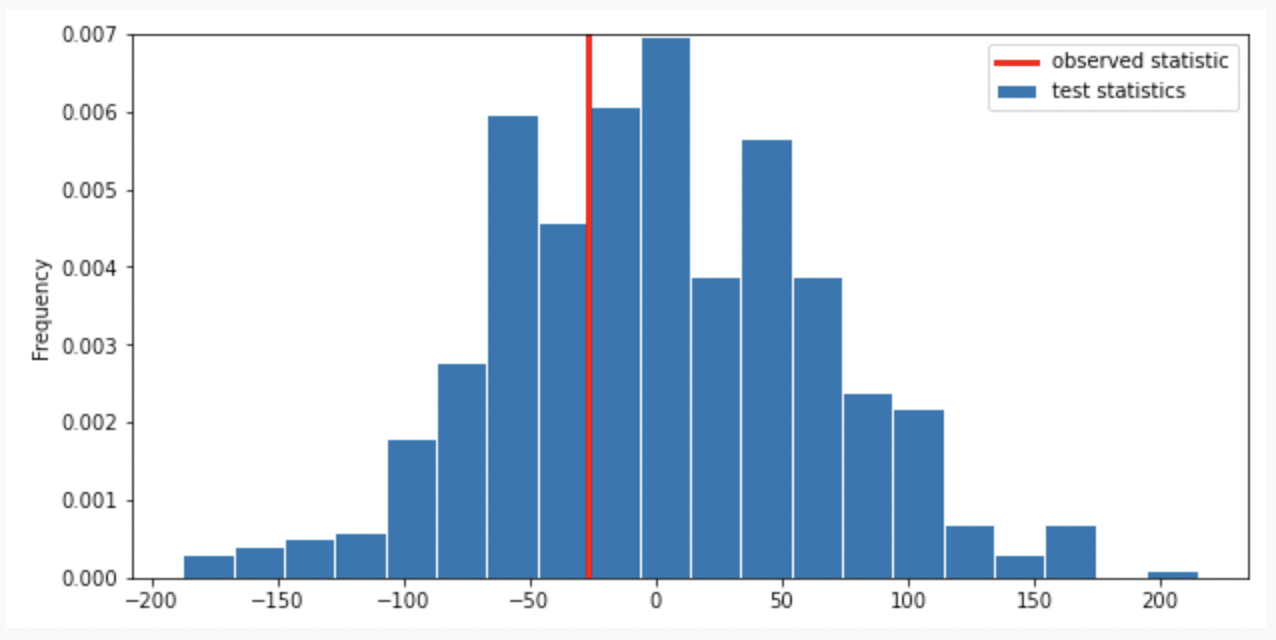Suppose our alternative hypothesis is that Chinstrap penguins weigh more on average than Adelie penguins. Which of the following is closest to the p-value for our hypothesis test?

• 0

• \frac{1}{4}

• \frac{1}{3}

• \frac{2}{3}

• \frac{3}{4}

• 1

Recall, the p-value is the chance, under the null hypothesis, that the test statistic is equal to the value that was observed in the data or is even further in the direction of the alternative. Thus, we compute the proportion of the test statistic that is equal or less than the observed statistic. (It is less than because less than corresponds to the alternative hypothesis “Chinstrap penguins weigh more on average than Adelie penguins”. Recall, when computing the statistic, we use Adelie’s mean mass minus Chinstrap’s mean mass. If Chinstrap’s mean mass is larger, the statistic will be negative, the direction of less than the observed statistic).

Thus, we look at the proportion of area less than or on the red line (which represents observed statistic), it is around \frac{1}{3}.

##### Difficulty: ⭐️⭐️

The average score on this problem was 80%.

## Problem 10

At the San Diego Model Railroad Museum, there are different admission prices for children, adults, and seniors. Over a period of time, as tickets are sold, employees keep track of how many of each type of ticket are sold. These ticket counts (in the order child, adult, senior) are stored as follows.

admissions_data = np.array([550, 1550, 400])

### Problem 10.1

Complete the code below so that it creates an array admissions_proportions with the proportions of tickets sold to each group (in the order child, adult, senior).

def as_proportion(data):
return __(a)__

admissions_proportions = as_proportion(admissions_data)

What goes in blank (a)?

Answer: data/data.sum()

To calculate proportion for each group, we divide each value in the array (tickets sold to each group) by the sum of all values (total tickets sold). Remember values in an array can be processed as a whole.

##### Difficulty: ⭐️

The average score on this problem was 95%.

### Problem 10.2

The museum employees have a model in mind for the proportions in which they sell tickets to children, adults, and seniors. This model is stored as follows.

model = np.array([0.25, 0.6, 0.15])

We want to conduct a hypothesis test to determine whether the admissions data we have is consistent with this model. Which of the following is the null hypothesis for this test?

• Child, adult, and senior tickets might plausibly be purchased in proportions 0.25, 0.6, and 0.15.

• Child, adult, and senior tickets are purchased in proportions 0.25, 0.6, and 0.15.

• Child, adult, and senior tickets might plausibly be purchased in proportions other than 0.25, 0.6, and 0.15.

• Child, adult, and senior tickets, are purchased in proportions other than 0.25, 0.6, and 0.15.

Answer: Child, adult, and senior tickets are purchased in proportions 0.25, 0.6, and 0.15. (Option 2)

Recall, null hypothesis is the hypothesis that there is no significant difference between specified populations, any observed difference being due to sampling or experimental error. So, we assume the distribution is the same as the model.

##### Difficulty: ⭐️⭐️

The average score on this problem was 88%.

### Problem 10.3

Which of the following test statistics could we use to test our hypotheses? Select all that could work.

• sum of differences in proportions

• sum of squared differences in proportions

• mean of differences in proportions

• mean of squared differences in proportions

• none of the above

Answer: sum of squared differences in proportions, mean of squared differences in proportions (Option 2, 4)

We need to use squared difference to avoid the case that large positive and negative difference cancel out in the process of calculating sum or mean, resulting in small sum of difference or mean of difference that does not reflect the actual deviation. So, we eliminate Option 1 and 3.

##### Difficulty: ⭐️⭐️

The average score on this problem was 77%.

### Problem 10.4

Below, we’ll perform the hypothesis test with a different test statistic, the mean of the absolute differences in proportions.

Recall that the ticket counts we observed for children, adults, and seniors are stored in the array admissions_data = np.array([550, 1550, 400]), and that our model is model = np.array([0.25, 0.6, 0.15]).

For our hypothesis test to determine whether the admissions data is consistent with our model, what is the observed value of the test statistic? Input your answer as a decimal between 0 and 1. Round to three decimal places. (Suppose that the value you calculated is assigned to the variable observed_stat, which you will use in later questions.)

We first calculate the proportion for each value in admissions_data \frac{550}{550+1550+400} = 0.22 \frac{1550}{550+1550+400} = 0.62 \frac{400}{550+1550+400} = 0.16 So, we have the distribution of the admissions_data

Then, we calculate the observed value of the test statistic (the mean of the absolute differences in proportions) \frac{|0.22-0.25|+|0.62-0.6|+|0.16-0.15|}{number\ of\ goups} =\frac{0.03+0.02+0.01}{3} = 0.02

##### Difficulty: ⭐️⭐️

The average score on this problem was 82%.

### Problem 10.5

Now, we want to simulate the test statistic 10,000 times under the assumptions of the null hypothesis. Fill in the blanks below to complete this simulation and calculate the p-value for our hypothesis test. Assume that the variables admissions_data, admissions_proportions, model, and observed_stat are already defined as specified earlier in the question.

simulated_stats = np.array([])
for i in np.arange(10000):
simulated_proportions = as_proportions(np.random.multinomial(__(a)__, __(b)__))
simulated_stat = __(c)__
simulated_stats = np.append(simulated_stats, simulated_stat)

p_value = __(d)__

What goes in blank (a)? What goes in blank (b)? What goes in blank (c)? What goes in blank (d)?

Answer: (a) admissions_data.sum() (b) model (c) np.abs(simulated_proportions - model).mean() (d) np.count_nonzero(simulated_stats >= observed_stat) / 10000

Recall, in np.random.multinomial(n, [p_1, ..., p_k]), n is the number of experiments, and [p_1, ..., p_k] is a sequence of probability. The method returns an array of length k in which each element contains the number of occurrences of an event, where the probability of the ith event is p_i.

We want our simulated_proportion to have the same data size as admissions_data, so we use admissions_data.sum() in (a).

Since our null hypothesis is based on model, we simulate based on distribution in model, so we have model in (b).

In (c), we compute the mean of the absolute differences in proportions. np.abs(simulated_proportions - model) gives us a series of absolute differences, and .mean() computes the mean of the absolute differences.

In (d), we calculate the p_value. Recall, the p_value is the chance, under the null hypothesis, that the test statistic is equal to the value that was observed in the data or is even further in the direction of the alternative. np.count_nonzero(simulated_stats >= observed_stat) gives us the number of simulated_stats greater than or equal to the observed_stat in the 10000 times simulations, so we need to divide it by 10000 to compute the proportion of simulated_stats greater than or equal to the observed_stat, and this gives us the p_value.

##### Difficulty: ⭐️⭐️

The average score on this problem was 79%.

### Problem 10.6

True or False: the p-value represents the probability that the null hypothesis is true.

• True

• False

Recall, the p-value is the chance, under the null hypothesis, that the test statistic is equal to the value that was observed in the data or is even further in the direction of the alternative. It only gives us the strength of evidence in favor of the null hypothesis, which is different from “the probability that the null hypothesis is true”.

##### Difficulty: ⭐️⭐️⭐️

The average score on this problem was 64%.

### Problem 10.7

The new statistic that we used for this hypothesis test, the mean of the absolute differences in proportions, is in fact closely related to the total variation distance. Given two arrays of length three, array_1 and array_2, suppose we compute the mean of the absolute differences in proportions between array_1 and array_2 and store the result as madp. What value would we have to multiply madp by to obtain the total variation distance array_1 and array_2? Input your answer below, rounding to three decimal places.